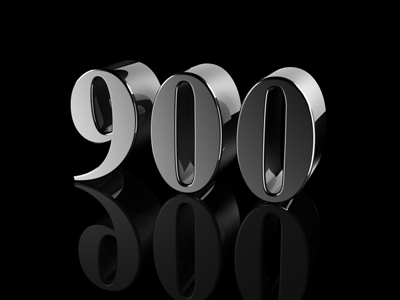What is 888 rounded to the nearest 100: 800 or 900?

# Rounding Numbers 2

This Math quiz is called 'Rounding Numbers 2' and it has been written by teachers to help you if you are studying the subject at elementary school. Playing educational quizzes is an enjoyable way to learn if you are in the 3rd, 4th or 5th grade - aged 8 to 11.

It costs only \$12.50 per month to play this quiz and over 3,500 others that help you with your school work. You can subscribe on the page at Join Us

Rounding numbers is usually to the nearest ten or hundred. You should now be pretty good at rounding. But, just to remind you if you've forgotten, here's how you do it:

When you round a number, you write it as an approximation of the original number: numbers can be rounded upwards and downwards. For example, 227 rounded to the nearest ten is 230 because 27 is closer to 30 than to 20. If you round 227 to the nearest hundred, it becomes 200 because 227 is nearer to 200 than to 300. All is well until someone asks you to round a number like 325 to the nearest ten: 25 is equidistant (the same distance) from 20 and 30. What do you do in such cases? You follow this RULE:

If the number that you use to decide whether to round upwards or downwards (let's call it the deciding number) is halfway or closer to the number to which you have to round to, round upwards BUT if it is less than halfway to the number to which you have to round to, round downwards.

Okay! Don't go round the bend - just round the numbers in this Math quiz!

1.
What is 142,785 rounded to the nearest 10,000?
150,000
140,000
142,000
143,000
42,785 is closer to 40,000 than to 50,000, so you round downwards
2.
What is 154 rounded to the nearest 100?
250
150
100
200
154 is closer to 200 than to 100, so you round upwards
3.
What is 34,562 rounded to the nearest 1,000?
35,000
34,000
34,600
35,500
4,562 is closer to 5,000 than to 4,000, so you round upwards
4.
What is 11,238 rounded to the nearest 100?
11,240
11,230
11,300
11,200
238 is closer to 200 than to 300, so you round downwards
5.
What is 63,836 rounded to the nearest 1,000?
63,000
64,800
64,000
63,800
3,836 is closer to 4,000 than to 3,000, so you round upwards
6.
What is 2,385 rounded to the nearest 10?
2,390
2,380
2,400
2,300
Although 85 is equidistant from 90 and 80, the rule means that you round upwards
7.
What is 486,385 rounded to the nearest 10,000?
490,000
496,000
480,000
486,000
86,385 is closer to 90,000 than to 80,000, so you round upwards
8.
What is 6,004 rounded to the nearest 10?
6,010
6,100
6,110
6,000
4 is closer to 0 than to 10, so you round downwards
9.
What is 63 rounded to the nearest 10?
80
70
60
50
63 is closer to 60 than to 70, so you round downwards
10.
What is 888 rounded to the nearest 100?
990
890
900
800
888 is closer to 900 than to 800, so you round upwards
Author:  Frank Evans